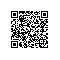# Day10 - JS 实现 Checkbox 中按住 Shift 的多选功能

## 项目效果## 操作方法

1. 选中 A 项
2. 按下 Shift
3. 再选中 B 项
4. A-B 之间的所有项都被选中或者取消

## 实现方法

### 方法一

Wes Bos 在文档里提供了一种解决办法：用一个变量，来标记这个范围。


const checkboxes = document.querySelectorAll('.inbox input[type="checkbox"]');

let startChecked;

//    处理方法一：用变量 inBetween 对需要选中的元素进行标记
function handleCheck0(e) {
let inBetween = false;
if(e.shiftKey && this.checked){
boxs.forEach(input => {
console.log(input);
if(input === startChecked || input ===this) {
inBetween = !inBetween;
}
if(inBetween) {
console.log("on");
input.checked = true;
}
});
}
startChecked = this;
}

checkboxes.forEach(checkbox => checkbox.addEventListener('click', handleCheck));


### 方法二


let startChecked;
let onOff = false;
//    处理方法二：新增onOff变量存储复选框将要改变的状态
function handleCheck2(e) {
let inBetween = false;
if (e.shiftKey) {
onOff = startChecked.checked ? true : false;
boxs.forEach(input => {
console.log(input);
if (input === startChecked || input === this) {
inBetween = !inBetween;
}
if (inBetween && input !== startChecked || input === this) {
input.checked = onOff;
}
});
startChecked = this;
}
startChecked = this;
}

onOff = startChecked.checked ? true : false; 根据startChecked设置要改变的状态。同时在if (inBetween && input !== startChecked || input === this)代码里面做了修改，新增了|| input === this，否则会出现最后一个的状态和其他复选框状态不一致的bug。

### 方法三

const boxArr = Array.from(boxs);
let startChecked;
let onOff = false;

// 处理方法二：利用数组索引获取需要选中的范围
function handleCheck1(e) {
if(!startChecked) startChecked = this;
onOff = startChecked.checked ? true : false;
if(e.shiftKey) {
let start = boxArr.indexOf(this);
let end = boxArr.indexOf(startChecked);
boxArr.slice(Math.min(start, end), Math.max(start, end) + 1)
.forEach(input => input.checked = onOff);
console.log(start + "+" + end);
}
startChecked = this;
}
1. 转换 Nodelist 为数组

const boxs = document.querySelectorAll('.inbox input[type="checkbox"]');
const boxArr = Array.from(boxs);
2. 针对按下了 Shift 键的情况，获取 A-B 范围

let start = boxArr.indexOf(this);
let end = boxArr.indexOf(startChecked);
3. 截取该范围内的数组元素，并改变选中状态

boxArr.slice(Math.min(start, end), Math.max(start, end) + 1)
.forEach(input => input.checked = onOff);
4. 确定选中 or 取消选中

onOff = startChecked.checked ? true : false;
5. 标记 A 值

if(!startChecked) startChecked = this;
/* ... */
startChecked = this;

## 源码下载

Github Source Code

## 春哥简介

github：http://github.com/liyuechun

## 技术交流

• 区块链技术交流QQ群：348924182
• 「区块链部落」官方公众号使用钉钉扫一扫加入圈子
+ 订阅